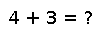# svds

``````..  svds(A; nsv=6, ritzvec=true, tol=0.0, maxiter=1000) -> (left_sv, s, right_sv, nconv, niter, nmult, resid)

``svds`` computes largest singular values ``s`` of ``A`` using Lanczos or Arnoldi iterations.
Uses :func:`eigs` underneath.

Inputs are:

* ``A``: Linear operator. It can either subtype of ``AbstractArray`` (e.g., sparse matrix) or duck typed. For duck typing ``A`` has to support ``size(A)``, ``eltype(A)``, ``A * vector`` and ``A' * vector``.
* ``nsv``: Number of singular values.
* ``ritzvec``: Whether to return the left and right singular vectors ``left_sv`` and ``right_sv``, default is ``true``. If ``false`` the singular vectors are omitted from the output.
* ``tol``: tolerance, see :func:`eigs`.
* ``maxiter``: Maximum number of iterations, see :func:`eigs`.

**Example**::

X = sprand(10, 5, 0.2)
svds(X, nsv = 2)``````

## Examples

Checking you are not a robot: